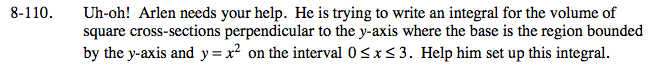### Home > CALC > Chapter 8 > Lesson 8.3.2 > Problem8-110

8-110.

Uh-oh! Arlen needs your help. He is trying to write an integral for the volume of square cross-sections perpendicular to the y-axis where the base is the region bounded by the y-axis and y = x2 on the interval 0 ≤ x ≤ 3. Help him set up this integral. Homework Help ✎These cross sections are squares. Their area formula is well known.

The function and the bounds must be in terms of y, because the cross sections are perpendicular to the y-axis. So, rewrite the function in terms of y and find the y-values that correspond with the given domain.